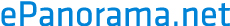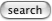# u-LAW and A-LAW definitions

A-law and u-law are companding schemes used in telephone network to get more dynamics to the 8 bit samples that is available with linear coding. Typically 12..14 bit samples (linear scale) sampled at 8 kHz sample are companded to 8 bit (logarithmic scale) for transmission over 64 kbit/s data channel. In the receiving end the data is then converter back to linear scale (12..14 bit) and played back. converted back

## u-law definition

u-LAW (pronounced mu-LAW) is

```          sgn(m)   (     |m |)       |m |
y=    ------- ln( 1+ u|--|)       |--| =< 1
ln(1+u)   (     |mp|)       |mp|
```
Another definition for mu-law I have seen
```                          ln(1+255 |x|)
output = sgn(x) ---------------------
ln(1+255)

x = normalized input ( between -1 and 1)
255 = compression parameter
sgn(x) = sign (+/-) of x

```

## a-law definition

A-LAW is
```     |     A    (m )                 |m |    1
|  ------- (--)                 |--| =< -
|  1+ln A  (mp)                 |mp|    A
y=|
| sgn(m) (        |m |)    1    |m |
| ------ ( 1+ ln A|--|)    - =< |--| =< 1
| 1+ln A (        |mp|)    A    |mp|
```

Values of u=100 and 255, A=87.6, mp is the Peak message value, m is the current quantised message value. (The formulae get simpler if you substitute x for m/mp and sgn(x) for sgn(m); then -1 <= x <= 1.)

Converting from u-LAW to A-LAW is in a sense "lossy" since there are quantizing errors introduced in the conversion.

"..the u-LAW used in North America and Japan, and the A-LAW used in Europe and the rest of the world and international routes.."

References:

• Modern Digital and Analog Communication Systems, B.P.Lathi., 2nd ed. ISBN 0-03-027933-X
• Transmission Systems for Communications, Fifth Edition, by Members of the Technical Staff at Bell Telephone Laboratories, Bell Telephone Laboratories, Incorporated, Copyright 1959, 1964, 1970, 1982